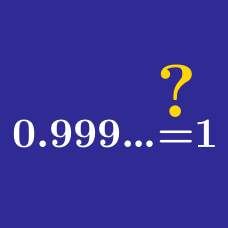Algebra

# Algebra Misconceptions

If $\frac{4}{2} > \frac{1}{a} ,$ is it always true that $4 \cdot a > 2 \cdot 1 ?$

Is it always true that $\large \frac{a}{b} + \frac{c}{d} = \frac{a+c}{b+d} ?$

$\frac{a}{bc} = \frac{a}{b} \cdot \frac{a}{c}$

For how many different values of $a$ is the above statement possibly true?

HINT: You should be trying to solve for $a ,$ so as a first step write the equation as $\frac{a}{bc} = \frac{a^2}{bc} .$

Which of these statements (if any) are $\color{#D61F06} { \text{false} }$?

Suppose you changed the rules of math so a negative times a negative was negative. So for instance $-3 \times -2 = -6$ instead of 6.

Which of these properties of arithmetic would no longer be true?

×